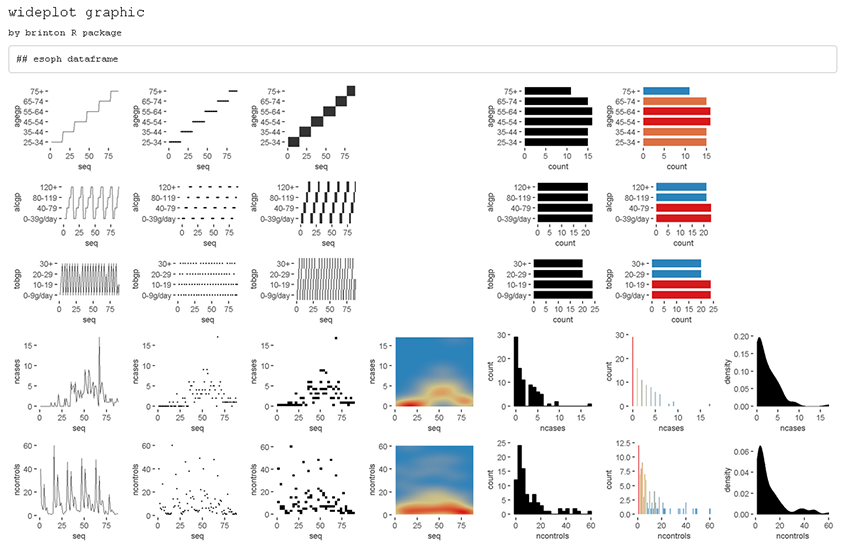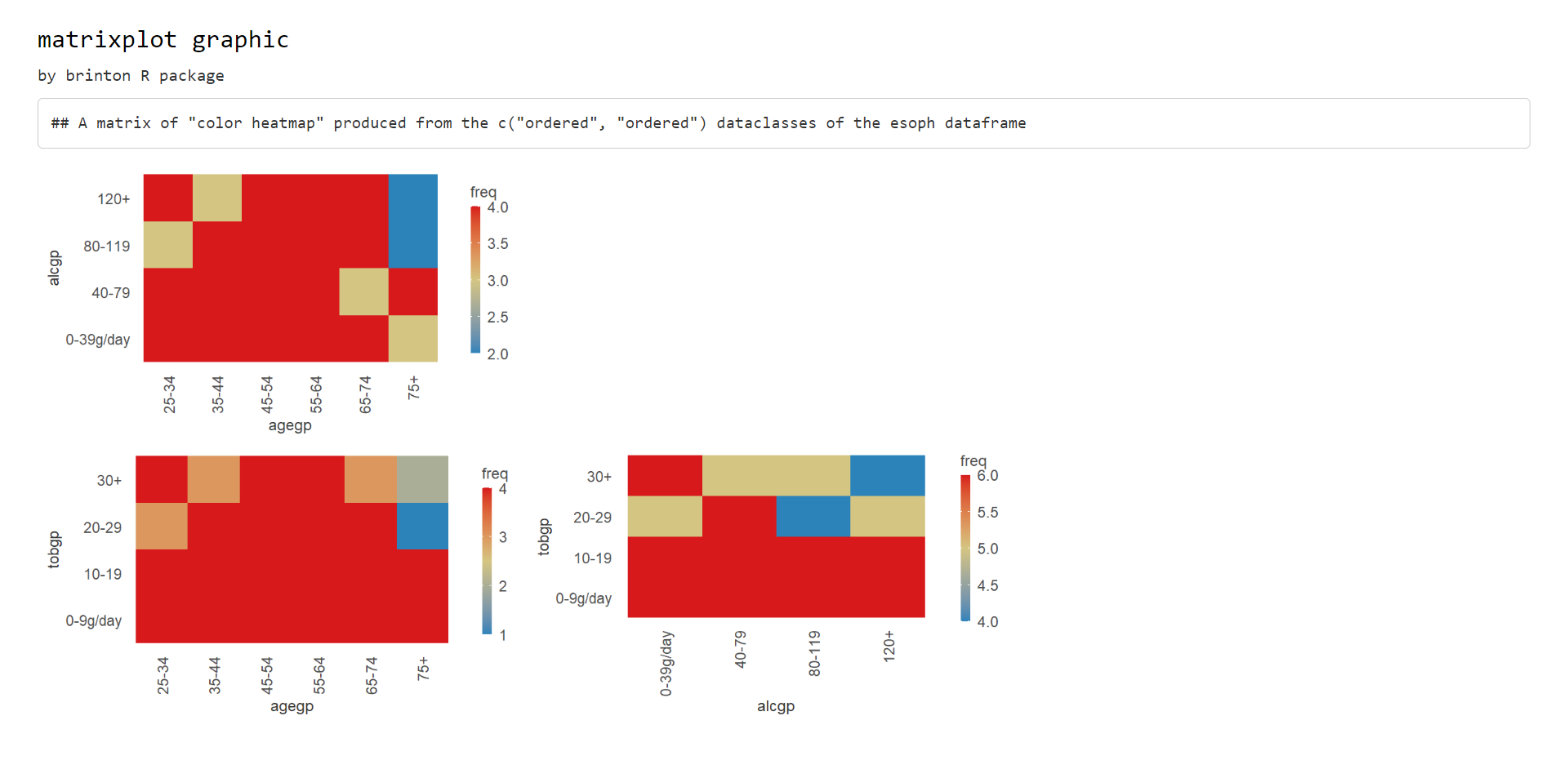NOTE: This vignette a short version, with the necessary updates, of the original article A Graphical EDA Tool with ggplot2: brinton published by The R Journal:

``````@article{RJ-2021-018,
author = {Pere Millán-Martínez and Ramon Oller},
title = {{A Graphical EDA Tool with ggplot2: brinton}},
year = {2021},
journal = {{The R Journal}},
doi = {10.32614/RJ-2021-018},
url = {https://doi.org/10.32614/RJ-2021-018},
pages = {311--320},
volume = {12},
number = {2}
}``````

# Introduction

We created `brinton` library to facilitate exploratory data analysis following the visual information-seeking mantra: “Overview first, zoom and filter, then details on demand”. The main idea is to assist the user during these three phases through four functions: `wideplot()`, `matrixplot()`, `longplot()` and `plotup()`. While each of these functions has its own arguments and purpose, all four serve to facilitate exploratory data analysis and the selection of a suitable graphic.

The library can be installed easily from the Comprehensive R Archive Network (CRAN) using the R console. When the library is loaded into memory, it provides a startup message that pays homage to Henry D. Hubbard’s enthusiastic introduction to the book Graphic Presentation by Willard Cope Brinton in 1939:

``````install.packages('brinton')
library(brinton)``````
``## M a G i C i N G R a P H S``

# The specimens

The `wideplot()`, `matrixplot()` and `plotup()` functions include arguments that allows the user to nominally plot specific graphics. The names and an example of each type of graphic are shown in the `1v specimen` and `2v specimen` vignettes dedicated to graphics that requiere one or two input variables. These graphics serve as an example so that the user can rapidly check whether a graphic has been incorporated, the type or types of variable for which it has been incorporated, and the label with which it has been identified. The suitability of a particular graphic will depend on the datasets of interest and the variables of each particular user.

In order to keep the package as compact as possible, these vignettes are not built whithin the package but they can be found at sciencegraph.github.io/brinton/articles/.

# brinton’s functions

The `wideplot()` function allows the user to explore a dataset as a whole using a grid of graphics in which each variable is represented through multiple graphics. Once we have explored the dataset as a whole, the `longplot()` allows us to explore other graphics for a given variable. This function also presents a grid of graphics, but instead of showing a selection of graphics for each variable, it presents the full array of graphics available in the library to represent a single variable. Once we have narrowed in on a certain graphic, we can use the `plotup()` function, which presents the values of a variable on a single graphic. We can access the code of the resulting graph and adapt it as needed. These three functions expand the graphic types that are presented automatically by the autoGEDA libraries in the R environment.

## The wideplot function

The `wideplot()` function returns a graphical summary of the variables included in the dataset to which it has been applied. First it groups the variables according to the following sequence: `logical`, `ordered`, `factor`, `character`, `datetime`, `numeric`. Next, it creates a multipanel graphic in html format, in which each variable of the dataset is represented in a row of the grid, while each column displays the different graphics possible for each variable. We called the resulting graphic type wideplot because it shows an array of graphics for all of the columns of the dataset. The structure of the function, the arguments it permits and its default values are as follows:

``````wideplot(data, dataclass = NULL, logical = NULL, ordered = NULL,
factor = NULL, character = NULL, datetime = NULL, numeric = NULL,
group = NULL, ncol = 7, label = 'FALSE')``````

The only argument necessary to obtain a result is `data` that expects a `data-frame` class object; `ncol` filters the first n columns of the grid, between 3 and 7, which will be shown. The fewer columns displayed, the larger the size of the resulting graphics, a feature that is especially useful if the scale labels dwarf the graphics area; `label` adds to the grid a vector below each group of rows according to the variable type, with the names and order of the graphics; `logical`, `ordered`, `factor`, `character`, `datetime` and `numeric` make it possible to choose which graphics appear in the grid and in what order, for each variable type. Finally, `group` changes the selection of graphics that are shown by default according to the criteria of the table 1.

The `wideplot()` function takes inspiration from this function, but instead of describing the dataset in textual or tabular form, it does it graphically (see figure 1). We can easily compare the results of these two functions, for example, with the dataset esoph from a case-control study of esophageal cancer in Ille-et-Vilaine, France. The dataset has three ordered factor-type variables and two numerical variables:

``str(esoph)``
``````## 'data.frame':    88 obs. of  5 variables:
##  \$ agegp    : Ord.factor w/ 6 levels "25-34"<"35-44"<..: 1 1 1 1 1 1 1 1 1 1 ...
##  \$ alcgp    : Ord.factor w/ 4 levels "0-39g/day"<"40-79"<..: 1 1 1 1 2 2 2 2 3 3 ...
##  \$ tobgp    : Ord.factor w/ 4 levels "0-9g/day"<"10-19"<..: 1 2 3 4 1 2 3 4 1 2 ...
##  \$ ncases   : num  0 0 0 0 0 0 0 0 0 0 ...
##  \$ ncontrols: num  40 10 6 5 27 7 4 7 2 1 ...``````
``wideplot(esoph)``Figure 1

If the order and graphic types to be shown for each variable type are not specified and if the graphic types aren’t filtered using the argument `group`, then the default graphic will contain an opinion-based selection graphics for each variable type, organized especially to facilitate comparison between graphics of the same row and between graphics of the same column. The user can overwrite this default selection of graphics as needed, using the arguments `logical`, `ordered`, `factor`, `character`, `datetime` and `numeric`.

group graphic.type
sequence includes the sequence in which the values are observed so that an axis develops this sequence. e.g., line graph, point-to-point graph
scatter marks represent individual observations. e.g., point graph, stripe graph
bin marks represent aggregated observations based on class intervals. e.g., histogram, bar graph
model represents models based on observations. e.g. density plot, violin plot
symbol represents models based on observations and not only points, lines or areas. e.g., box plo.
GOF represents the goodness of fit of some values with respect to a model. e.g. qq plot
random chosen at random

## The matrixplot function

We place the `matrixplot()` function as a second step right after understanding the structure of the dataset using the `wideplot()` function.

The `matrixplot()` function produces a variant of a type of plot known as a pairs plot that is intended to look at the interrelationships between pairs of variables (or in other words bivariate analysis) and thus raise the level of analysis. The bivariate analysis allows, for example, to observe if two variables are correlated, to quantify that correlation, to compare the distribution of two or more groups or to make counts with respect to pairs of observations (bivariate frequencies). It creates a multipanel graphic in html format, in which each panel represents the relations between the values of two variables. The structure of the function, the arguments it permits and its default values are as follows:

``matrixplot(data, dataclass = NULL, diagram = NULL``

The `dataclass` argument expects a vector with the types of variables to be combined in each panel of the matrixplot output. Depending on whether this argument contains a single type of variable or two different types, the function produces arrays of graphs with different characteristics as detailed below.

### Two different outputs

A first scenario occurs if the pairs of variables that combine each panel of the array are of the same class. This scenario can occur in three different circumstances: if the argument `dataclass` is a null vector, then the argument defaults to the value `dataclass = c('numeric', 'numeric')`; if the argument is not null but is a vector of length 1, for example `'ordered'`, the pairs of variables will be between variables of this same specified class, in this case it would be `dataclass = c('ordered', 'ordered ')`; if the argument is a vector of length 2 with the same variable class repeated, for example `dataclass = c('factor', 'factor')`.

When this first scenario occurs, an output could have been a classic square matrix, in which the diagonal shows the names of the variables like the one in the figure @ref(fig:pairsplot). The output, however, obtained by the `matrixplot()` function, is the matrix containing only the cells below the leading diagonal, and combining only the variables of the data set of the class specified by the `dataclass` argument (see figure 2).

``matrixplot(esoph, dataclass = c("ordered"), diagram = "color heatmap")``Figure 2

The second scenario occurs if the pairs of variables that are combined in each panel of the graph are of different classes. This scenario can only occur when the `dataclass` argument is a vector of length 2 with two different variable classes, eg `dataclass = c('numeric', 'factor')`. In this case, the resulting graph matrix is a rectangular matrix in which the columns represent variables of one type and the rows of the other type (see figure 3).

``matrixplot(esoph, dataclass = c("numeric", "ordered"), diagram = c("box plot"))``